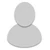## FANDOM

26,105 Pages

• So.... the "Finding mass from a size change"-section in the Inverse Square Law Page has a little problem. That problem being that it is wrong as far as I see.

Scaling the size of an 3D object follows a cube law, not an inverse square law.

That is to say, if something grows x times larger (in length, width and height), than its volume becomes x^3 time larger and, if the density stays constant, its mass increases by the factor x^3.

To show based on the example on the page: If you have a sphere that is 1m in diameter that ways 3000kg, then its volume is 4/3*pi*(r)^3 = 4/3*pi*(1/2)^3 = 0.5235987755982989 m^3 and its density is 3000kg / (0.5235987755982989) m^3 = 5729.577951308231793071 kg/m^3.

If you now increase the spheres diameter to 10 m, while keeping the density constant, the volume of the new sphere is 4/3*pi*(10/2)^3 = 523.59877559 m^3 = 10^3 * 0.52359877559 m^3 and hence its mass is 5729.577951308231793071 kg/m^3 * 523.59877559 m^3 = 3 000 000 kg = 10^3 * 3000kg. So you scaled it up by 10 and the volume and mass became 10^3 times larger.

Sooo... considering that this is no inverse square law we should delete that section from the page. If calculations used that formula they would have to get fixed.

• I always found strange that people called inverse square law when mass was the one being found. Yes, those rules are different.

• Actually yes it's called the cube-square law which has nothing to do with the inverse square law.  Should we make a cube-square law page for this?

I guess, I will take that section from the page for now.

If someone is willing to write it one could add an explanation about how volume is cubed when its scaled seomwhere.

If it's long as a separate page or if it's short on the calculations page in the volume section.

(we should probably restructure those sections sometime...)

•